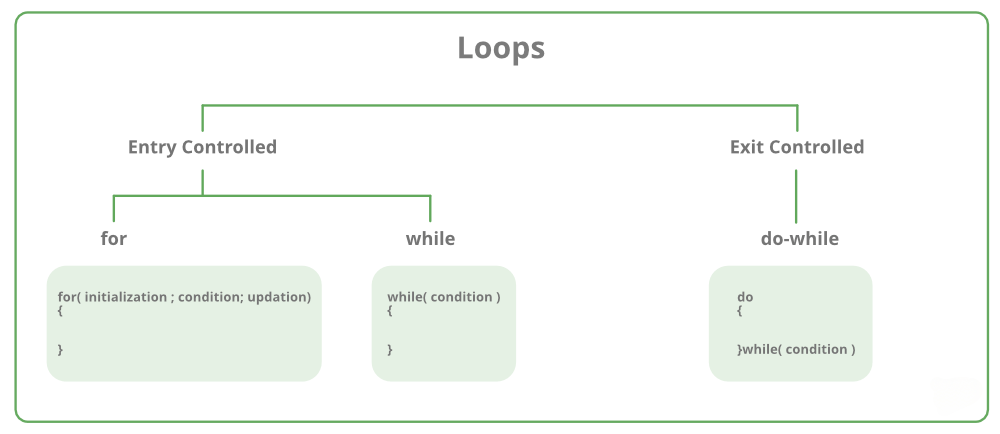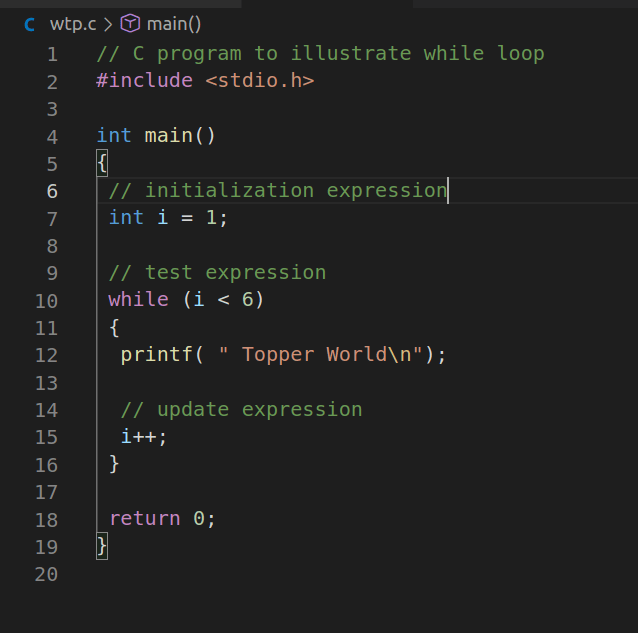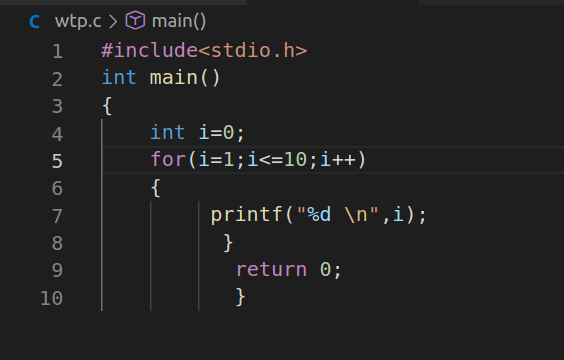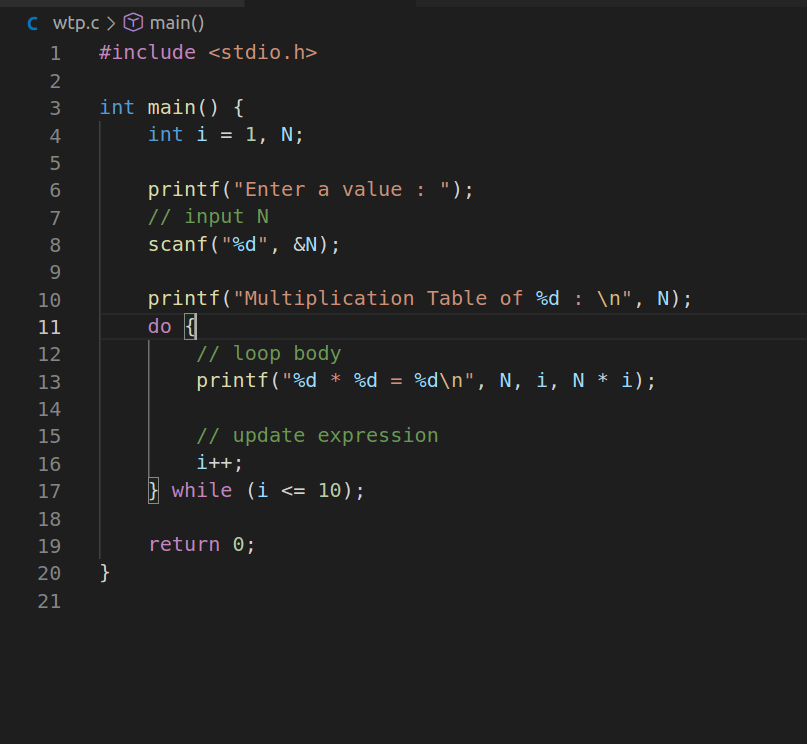# loops in c language

During programming, sometimes we might need to execute a certain code statement again and again. We can write the code statement as many times as we need it to execute but that would be very inefficient, because what if you want a code statement to execute a 100 times . This is why we use loops.In any programming language including C language, loops are used to execute a single statement or a set of statements, repeatedly, until a particular condition is satisfied.

Types Of Loop In C

There are 3 types of Loops in C language

### 2 . for loop

3 . do while loop##### 1. While Loop

The while loop is an entry controlled loop. It is completed in 3 steps.

a. Variable initialization.

(e.g int x = 0;)                                                                                                    b. condition

(e.g while(x <= 10))                                                                                              c. Variable increment or decrement ( x++ or x– or x = x + 2 )

### Syntax Of While Loop :

variable initialization;                                                                         while(condition)                                      {

//   statements;                                  // variable increment or decrement;

}

Examples :##### 2. For Loop

A for loop is a repetition control structure that allows us to write a loop that is executed a specific number of times.The loop enables us to perform n number of steps together in one line.

Syntax:

for (initialization expr; test expr; update expr)                                            {                                                             // body of the loop                                                                                                                                                         // statements we want to execute

}

Initialization Expression:      In this expression, we have to initialize the loop counter to some value. for example: int i=1;     Test   Expression:       In this expression, we have to test the condition. If the condition evaluates to true then we will execute the body of the loop and go to update expression otherwise we will exit from the for a loop. For example: i <= 10;           Update  Expression:  After executing the loop body this expression increments/decrements the loop variable by some value. for example: i++;

Examples:##### 3. Do While Loop

In do-while loops also the loop execution is terminated on the basis of test conditions. The main difference between a do-while loop and the while loop is in the do-while loop the condition is tested at the end of the loop body, i.e do-while loop is exit controlled whereas the other two loops are entry controlled loops. Note: In a do-while loop, the loop body will execute at least once irrespective of the test condition.

Syntax:

initialization expression;

do

{

// statements update_expression;

}

while (test_expression);

Examples: